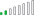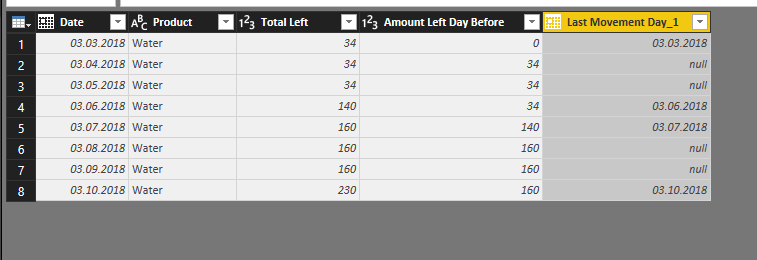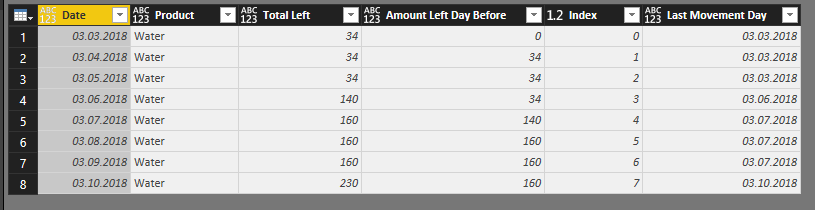cancel
Showing results for
Did you mean:Helper I

## Repeat last date for each row

Hello everyone,

As you can see, i need a calculated column, that repeats the last date whenever the value between "Total Left" and "Amount Left Day Before" doesnt change, and if i get a difference i get the present day.

So, for "Date" 03/03/18 i got 34 and 0.... so it returns 03/03/2018. The next day i still got 34 and 34, so it keeps the date 03/03/18.

However on date 03/06/18 i got 140 and 34, so the "Last Movement Day" receive 03/06/18.

I did a calculated column, but i cant keep the previous day ( i got a blank value ) when "amounts" column keeps equals. Can someone help me filling blank cells with present date values?

Many thanks !!

 ( i need that columm ) (best what i got ) Date Product Total Left Amount Left Day Before Last Movement Day Last Movement Day 03/03/2018 Water 34 0 03/03/2018 03/03/2018 03/04/2018 Water 34 34 03/03/2018 (blank) 03/05/2018 Water 34 34 03/03/2018 (blank) 03/06/2018 Water 140 34 03/06/2018 03/06/2018 03/07/2018 Water 160 140 03/07/2018 03/07/2018 03/08/2018 Water 160 160 03/07/2018 (blank) 03/09/2018 Water 160 160 03/07/2018 (blank) 03/10/2018 Water 230 160 03/10/2018 03/10/2018
1 ACCEPTED SOLUTIONCommunity Champion

@brunomoriya

here is a dax calculated column

```Column =
VAR Dif = Table1[Total Left] - Table1[Amount Left Day Before]
VAR previousrow =
TOPN (
1,
FILTER (
Table1,
Table1[Product] = EARLIER ( Table1[Product] )
&& Table1[Date] < EARLIER ( Table1[Date] )
&& ( Table1[Total Left] - Table1[Amount Left Day Before] )
<> 0
),
[Date], DESC
)
RETURN
IF ( Dif = 0, MINX ( previousrow, [Date] ), Table1[Date] )```

Regards
Zubair

4 REPLIES 4Resolver II

What I would suggest in your case is simply apply "Fill down" function.

Before:Select the last column and click on Fill and down:What I get:Let me know if this is the solution you are after. If not, we will work it out in Power Query - no problem 🙂

ThanksResolver II

Ok, I think I might have jumped to gun here. I see that you are using a calculated column, so Fill Down function will not work.

My result:The code I have used:

```let
Source = Excel.Workbook(File.Contents("C:\PowerBI_problem.xlsx"), null, true),
RFC_Sheet = Source{[Item="Sheet1",Kind="Sheet"]}[Data],
New_app = List.Accumulate(#"Added Index"[Index],{0}, (state,current) =>  if current = 0 then {#"Added Index"{0}[Date]} else if #"Added Index"{current-1}[Total Left] = #"Added Index"{current}[Total Left] then state & {List.Last(state)} else state & {#"Added Index"{current}[Date]}),
//Convert each row to a list
Column_rename_torows = Table.ToRows(Column_rename),
//Rename it using the list

in
Renamed_cols```

I have pasted your table into Excel and then imported into Power BI into Power Query:` #"Added Index" = Table.AddIndexColumn(#"Removed Bottom Rows", "Index", 0, 1)`

``` New_app = List.Accumulate(#"Added Index"[Index],{0}, (state,current) =>  if current = 0 then {#"Added Index"{0}[Date]} else if #"Added Index"{current-1}[Total Left] = #"Added Index"{current}[Total Left] then state & {List.Last(state)} else state & {#"Added Index"{current}[Date]}),
```

I have explained it here.  The effect is the list of values you are after:The rest of the steps involves pasting it all together and renaming the columns:

```    Add_columns= Table.FromColumns(Table.ToColumns(#"Added Index")&{New_app}),
//Convert each row to a list
Column_rename_torows = Table.ToRows(Column_rename),
//Rename it using the list

Let me know if it helped.

ThanksCommunity Champion

@brunomoriya

here is a dax calculated column

```Column =
VAR Dif = Table1[Total Left] - Table1[Amount Left Day Before]
VAR previousrow =
TOPN (
1,
FILTER (
Table1,
Table1[Product] = EARLIER ( Table1[Product] )
&& Table1[Date] < EARLIER ( Table1[Date] )
&& ( Table1[Total Left] - Table1[Amount Left Day Before] )
<> 0
),
[Date], DESC
)
RETURN
IF ( Dif = 0, MINX ( previousrow, [Date] ), Table1[Date] )```

Regards
ZubairHelper I

Thanks a lot !! This worked !!

@Michal_cwiok

Thanks for helping, but a dax solution is a better way for me!!Announcements#### Exclusive opportunity for Women!

Join us for a free, hands-on Microsoft workshop led by women trainers for women where you will learn how to build a Dashboard in a Day!#### Power Platform Conference-Power BI and Fabric Sessions

Join us Oct 1 - 6 in Las Vegas for the Microsoft Power Platform Conference.Top Solution Authors
Top Kudoed Authors
Users online (3,840)